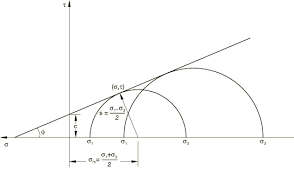## How to Calculate and Solve for Mohr – Coulomb Criterion | Rock MechanicsThe image above represents mohr – coulomb criterion.

To compute for mohr – coulomb criterion, three essential parameters are needed and these parameters are Cohesion (|τo), Co-efficient of Friction (μ) and Normal Stress (σn).

The formula for calculating the mohr – coulomb criterion:

|τ| = τo + μσn

Where;

|τ = Mohr – Coulomb Criterion
o = Cohesion
μ = Co-efficient of Friction
σn = Normal Stress

Let’s solve an example;
Find the mohr – coulomb criterion when the cohesion is 7, the co-efficient of friction is 30 and the normal stress is 28.

This implies that;

o = Cohesion = 7
μ = Co-efficient of Friction = 30
σn = Normal Stress = 28

|τ| = τo + μσn
|τ| = 7 + 30(28)
|τ| = 7 + 840
|τ| = 847

Therefore, the mohr – coulomb criterion is 847.

Calculating the Cohesion when the Mohr – Coulomb Criterion, the Co-efficient of Friction and the Normal Stress is Given.

o = |τ|  – μσn

Where;

o = Cohesion
|τ = Mohr – Coulomb Criterion
μ = Co-efficient of Friction
σn = Normal Stress

Let’s solve an example;
Find the cohesion when the mohr – coulomb criterion is 44, the co-efficient of friction is 10 and the normal stress is 4.

This implies that;

|τ = Mohr – Coulomb Criterion = 44
μ = Co-efficient of Friction = 10
σn = Normal Stress = 4

o = |τ|  – μσn
o = 44  – 10(4)
o = 44  – 40
o = 4

Therefore, the cohesion is 4.